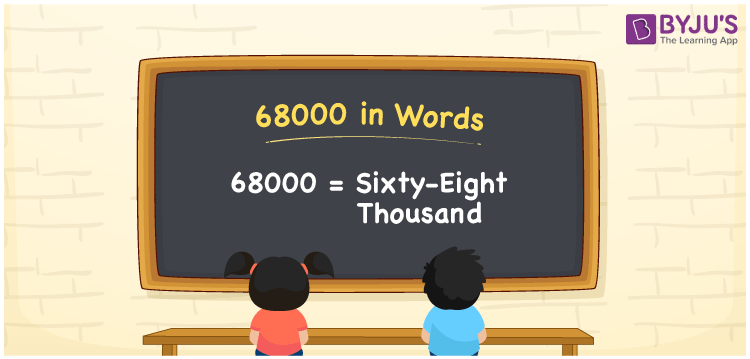# 68000 in Words

68000 in words is written as Sixty-eight thousand. In both the International System of Numerals and the Indian System of Numerals, 68000 is written as Sixty-eight thousand. The number 68000 is a Cardinal Number as it represents some quantity. For example, “the Smart-TV costs 68000 rupees”, 68000 represents the cost of the TV.

 68000 in words Sixty-eight thousand Sixty-eight thousand in number 68000

## 68000 in English Words

68000 in English words is read as “Sixty-eight thousand.”## How to Write 68000 in Words?

To write 68000 in words, we shall use the place value chart. In the place value chart, put 6 in the ten thousands, 8 in the thousands, and 0 in the hundreds, the tens and the ones place, respectively. Let us make a place value chart to write the number 68000 in words.

 Ten Thousands Thousands Hundreds Tens Ones 6 8 0 0 0

Thus, we can write the expanded form as

6 × Ten Thousand + 8 × Thousand + 0 × Hundred + 0 × Ten + 0 × One

= 6 × 10000 + 8 × 1000 + 0 × 100 + 0 × 10 + 0 × 1

= 60000 + 8000 + 0 + 0 + 0

= 68000

= Sixty-eight thousand.

68000 is a natural number, the successor of 67999 and the predecessor of 68001.

68000 in words – Sixty-eight thousand

• Is 68000 an odd number? – No
• Is 68000 an even number? – Yes
• Is 68000 a perfect square number? – No
• Is 68000 a perfect cube number? – No
• Is 68000 a prime number? – No
• Is 68000 a composite number? – Yes

## Frequently Asked Questions on 68000 in Words

Q1

### How to write 68000 in words?

68000 in words is written as Sixty-eight thousand.
Q2

### How to write 68000 in the International and Indian System of Numerals?

In both, the system of numerals, 68000 in words, is written as Sixty-eight thousand.
Q3

### How to write 68000 in a place value chart?

In the place value chart, write 6 in the ten thousands place, 8 in the thousands, and 0 in the hundreds, the tens and the ones place respectively.